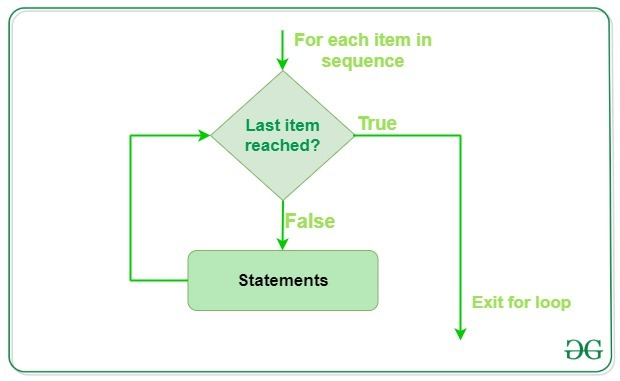# Python For Loops

• Difficulty Level : Easy
• Last Updated : 14 Jul, 2022

Python For loop is used for sequential traversal i.e. it is used for iterating over an iterable like String, Tuple, List, Set or Dictionary.

In Python, there is no C style for loop, i.e., for (i=0; i<n; i++). There is “for” loop which is similar to each loop in other languages. Let us learn how to use for in loop for sequential traversals

Note: In Python, for loops only implements the collection-based iteration.

### For Loops Syntax

```for var in iterable:
# statements```

## Flowchart of for loopHere the iterable is a collection of objects like lists, tuples. The indented statements inside the for loops are executed once for each item in an iterable. The variable var takes the value of the next item of the iterable each time through the loop.

## Python3

 `# Python program to illustrate``# Iterating over a list``l ``=` `[``"geeks"``, ``"for"``, ``"geeks"``]``for` `i ``in` `l:``    ``print``(i)`

Output:

```Geeks
for
geeks```

## Python3

 `# Iterating over dictionary``print``(``"Dictionary Iteration"``)``d ``=` `dict``()``d[``'xyz'``] ``=` `123``d[``'abc'``] ``=` `345``for` `i ``in` `d:``    ``print``(``"% s % d"` `%` `(i, d[i]))`

Output:

```Dictionary Iteration
xyz  123
abc  345```

## Python3

 `# Iterating over a String``print``(``"String Iteration"``)``s ``=` `"Geeks"``for` `i ``in` `s:``    ``print``(i)`

Output:

```String Iteration
G
e
e
k
s```

## Loop Control Statements

Loop control statements change execution from its normal sequence. When execution leaves a scope, all automatic objects that were created in that scope are destroyed. Python supports the following control statements.

### Continue Statement in Python

Python continue Statement returns the control to the beginning of the loop.

## Python3

 `# Prints all letters except 'e' and 's'``for` `letter ``in` `'geeksforgeeks'``:``    ``if` `letter ``=``=` `'e'` `or` `letter ``=``=` `'s'``:``        ``continue``    ``print``(``'Current Letter :'``, letter)`

Output:

```Current Letter : g
Current Letter : k
Current Letter : f
Current Letter : o
Current Letter : r
Current Letter : g
Current Letter : k```

### Break Statement in Python

Python break statement brings control out of the loop.

## Python3

 `for` `letter ``in` `'geeksforgeeks'``:` `    ``# break the loop as soon it sees 'e'``    ``# or 's'``    ``if` `letter ``=``=` `'e'` `or` `letter ``=``=` `'s'``:``        ``break` `print``(``'Current Letter :'``, letter)`

Output:

`Current Letter : e`

### Pass Statement in Python

The pass statement to write empty loops. Pass is also used for empty control statements, functions, and classes.

## Python3

 `# An empty loop``for` `letter ``in` `'geeksforgeeks'``:``    ``pass``print``(``'Last Letter :'``, letter)`

Output:

`Last Letter : s `

## range() function in Python

Python range() is a built-in function that is used when a user needs to perform an action a specific number of times. range() in Python(3.x) is just a renamed version of a function called xrange() in Python(2.x).

The range() function is used to generate a sequence of numbers. Depending on how many arguments the user is passing to the function, user can decide where that series of numbers will begin and end as well as how big the difference will be between one number and the next.range() takes mainly three arguments.

• start: integer starting from which the sequence of integers is to be returned
• stop: integer before which the sequence of integers is to be returned.
The range of integers end at stop – 1.
• step: integer value which determines the increment between each integer in the sequence

## Python3

 `# Python Program to``# show range() basics` `# printing a number``for` `i ``in` `range``(``10``):``    ``print``(i, end``=``" "``)` `# performing sum of first 10 numbers``sum` `=` `0``for` `i ``in` `range``(``1``, ``10``):``    ``sum` `=` `sum` `+` `i``print``(``"\nSum of first 10 numbers :"``, ``sum``)`

Output:

```0 1 2 3 4 5 6 7 8 9
Sum of first 10 numbers : 45```

## For loop in Python with else

In most programming languages (C/C++, Java, etc), the use of else statements has been restricted with the if conditional statements. But Python also allows us to use the else condition with for loops.

Note: The else block just after for/while is executed only when the loop is NOT terminated by a break statement

## Python3

 `# Python program to demonstrate``# for-else loop` `for` `i ``in` `range``(``1``, ``4``):``    ``print``(i)``else``:  ``# Executed because no break in for``    ``print``(``"No Break\n"``)`

Output:

```1
2
3
No Break```

Note: For more information refer to our Python for loop with else tutorial.

My Personal Notes arrow_drop_up